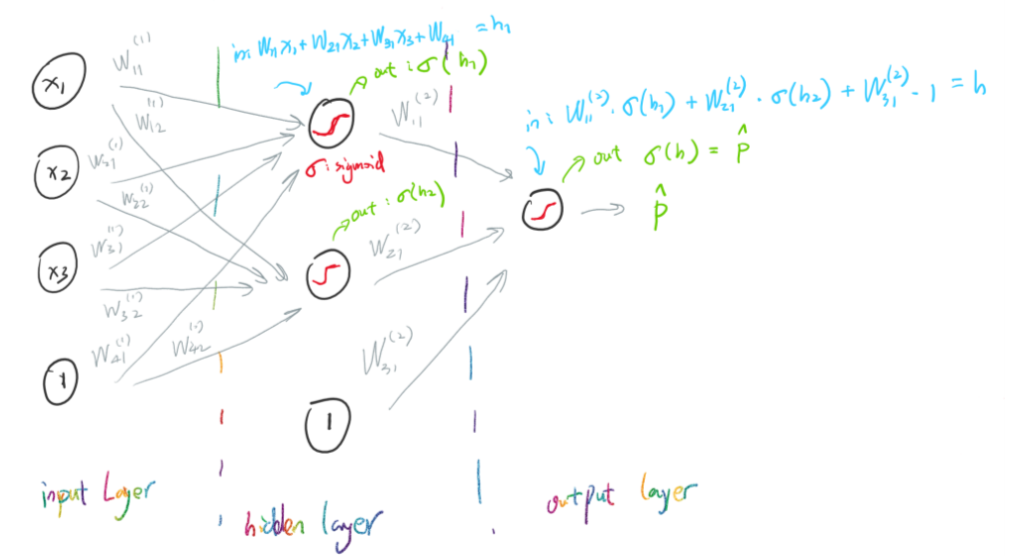# Chapter 8 – Feedforward#

Data Science and Machine Learning for Geoscientists

Let’s take a look at how feedforward is processed in a three layers neural net.Figure 8.1

From the figure 8.1 above, we know that the two input values for the first and the second neuron in the hidden layer are

(46)#$h_1^{(1)} = w_{11}^{(1)}*x_1 + w_{21}^{(1)}*x_2 + w_{31}^{(1)}*x_3+ w_{41}^{(1)}*1$
(47)#$h_2^{(1)} = w_{12}^{(2)}*x_1 + w_{22}^{(2)}*x_2 + w_{32}^{(2)}*x_3+ w_{42}^{(2)}*1$

where the $$w^{(n)}_{4m}$$ term is the bias term in the form of weight.

To simplify the two equations above, we can use matrix

(48)#$\begin{split} H^{(1)} = [h_1^{(1)} \;\; h_2^{(1)}] = [x_1 \;\; x_2 \;\; x_3 \;\; 1] \begin{bmatrix} w^{(1)}_{11} & w^{(1)}_{12} \\ w^{(1)}_{21} & w^{(1)}_{22} \\ w^{(1)}_{31} & w^{(1)}_{32} \\ w^{(1)}_{41} & w^{(1)}_{4 2} \end{bmatrix} \end{split}$

Similarly, the two outputs from the input layer can be the inputs for the hidden layer

(49)#$\sigma(H^{(1)}) = [\sigma(h_1^{(1)}) \;\; \sigma( h_2^{(1)})]$

This in turns can be the input values for the next layer (output layer)

(50)#$h^{(2)} = w^{(2)}_{11}* \sigma(h^{(1)}_1)+w^{(2)}_{21} *\sigma(h^{(1)}_2)+w^{(2)}_{31}*1$

Again, we can simplify this equation by using matrix

(51)#$\begin{split} H^{(2)} = [\sigma(h_1^{(1)}) \;\;\sigma(h_2^{(1)}) \; \; 1] \begin{bmatrix} w^{(2)}_{11} \\ w^{(2)}_{21} \\ w^{(2)}_{31} \end{bmatrix} \end{split}$

Then we send this value $$h^{(2)}$$ into the sigma function in the final output layer to obtain the prediction

(52)#$\hat{y} = \sigma(h^{(2)})$

To put all the equation of three layers together, we can have

(53)#$\begin{split} \hat{y} = \sigma(\sigma([x_1 \;\; x_2 \;\; x_3 \;\; 1] \begin{bmatrix} w^{(1)}_{11} & w^{(1)}_{12} \\ w^{(1)}_{21} & w^{(1)}_{22} \\ w^{(1)}_{31} & w^{(1)}_{32} \\ w^{(1)}_{41} & w^{(1)}_{42} \end{bmatrix}) \begin{bmatrix} w^{(2)}_{11} \\ w^{(2)}_{21} \\ w^{(2)}_{31} \end{bmatrix}) \end{split}$

Or we can simplify it to be

(54)#$\hat{y} = \sigma(\sigma(xW^{(1)})W^{(2)})$

This is the feedforward process: based on the known weights $$W$$ and input $$x$$ to calculate the prediction $$\hat{y}$$.

Finally, it’s easy to write code computing the output from a Network instance. We begin by defining the sigmoid function:

def sigmoid(z):
return 1.0/(1.0+np.exp(-z))


Note that when the input z is a vector or Numpy array, Numpy automatically applies the function sigmoid elementwise, that is, in vectorized form.

We then add a feedforward method to the Network class, which, given an input a for the network, returns the corresponding output:

def feedforward(self, a):
"""Returning the output a, which is the input to the next layer"""
for b, w in zip(self.biases, self.weights):
a = sigmoid(np.dot(w, a)+b)
return a